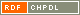# Show document

Title: Identities with generalized skew derivations on Lie ideals De Filippis, Vincenzo (Author)Fošner, Ajda (Author)Wei, Feng (Author)http://dx.doi.org/10.1007/s10468-012-9344-4 English Not categorized 1.01 - Original Scientific Article IAM - Andrej Marušič Institute Let ▫$m, n$▫ be two nonzero fixed positive integers, ▫$R$▫ a 2-torsion free prime ring with the right Martindale quotient ring ▫$Q$▫, ▫$L$▫ a non-central Lie ideal of ▫$R$▫, and ▫$\delta$▫ a derivation of ▫$R$▫. Suppose that ▫$\alpha$▫ is an automorphism of ▫$R$▫, ▫$D$▫ a skew derivation of ▫$R$▫ with the associated automorphism ▫$\alpha$▫, and ▫$F$▫ a generalized skew derivation of ▫$R$▫ with the associated skew derivation ▫$D$▫. If ▫$$F(x^{m+n}) = F(x^m)x^n + x^m \delta (x^n)$$▫ is a polynomial identity for ▫$L$▫, then either ▫$R$▫ satisfies the standard polynomial identity ▫$s_4(x_1, x_2, x_3, x_4)$▫ of degree 4, or ▫$F$▫ is a generalized derivation of ▫$R$▫ and ▫$\delta = D$▫. Furthermore, in the latter case one of the following statements holds: (1) ▫$D = \delta = 0$▫ and there exists ▫$a \in Q$▫ such that ▫$F(x) = ax$▫ for all ▫$x \in R$▫; (2) ▫$\alpha$▫ is the identical mapping of ▫$R$▫. mathematics, algebra, polynomial identity, generalized skew derivation, prime ring 2013 str. 1017-1038 Vol. 16, issue 4 1386-923X 512.552 166536571745 79Document is not linked to any category.

Average score: (0 votes) Voting is allowed only to logged in users.Hover the mouse pointer over a document title to show the abstract or click on the title to get all document metadata.

## Secondary language

Language: Slovenian V članku je obravnavana posebna identiteta na Liejevem idealu prakolobarja, ki vklučuje posplošeno ▫$\alpha$▫-odvajanje. matematika, algebra, polinomska identiteta, prakolobar, posplošeno ▫\$\alpha▫-odvajanje